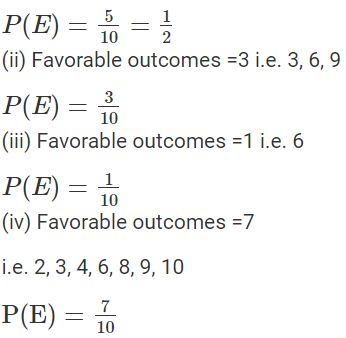# Selina Solutions Concise Maths Class 8 Chapter 23: Probability

Selina Solutions Concise Maths Class 8 Chapter 23 Probability is one of the important chapters as it would be continued in higher levels of education. For this purpose, it is necessary for the students to understand each and every concept covered in this chapter clearly. Students will be able to cross check their answers while solving the textbook questions and understand the topics which they are weak at. Here, the students can download the ICSE Selina Solutions Class 8 Maths Chapter 23 Probability PDF, from the link which is given below.

Probability is a branch of Mathematics which is concerned with how likely an event is to occur or if that proposition is true. Students who aspire to perform well in the Class 8 exams are advised to solve the problems on a daily basis. It will also help them to clear their queries on time without any confusion.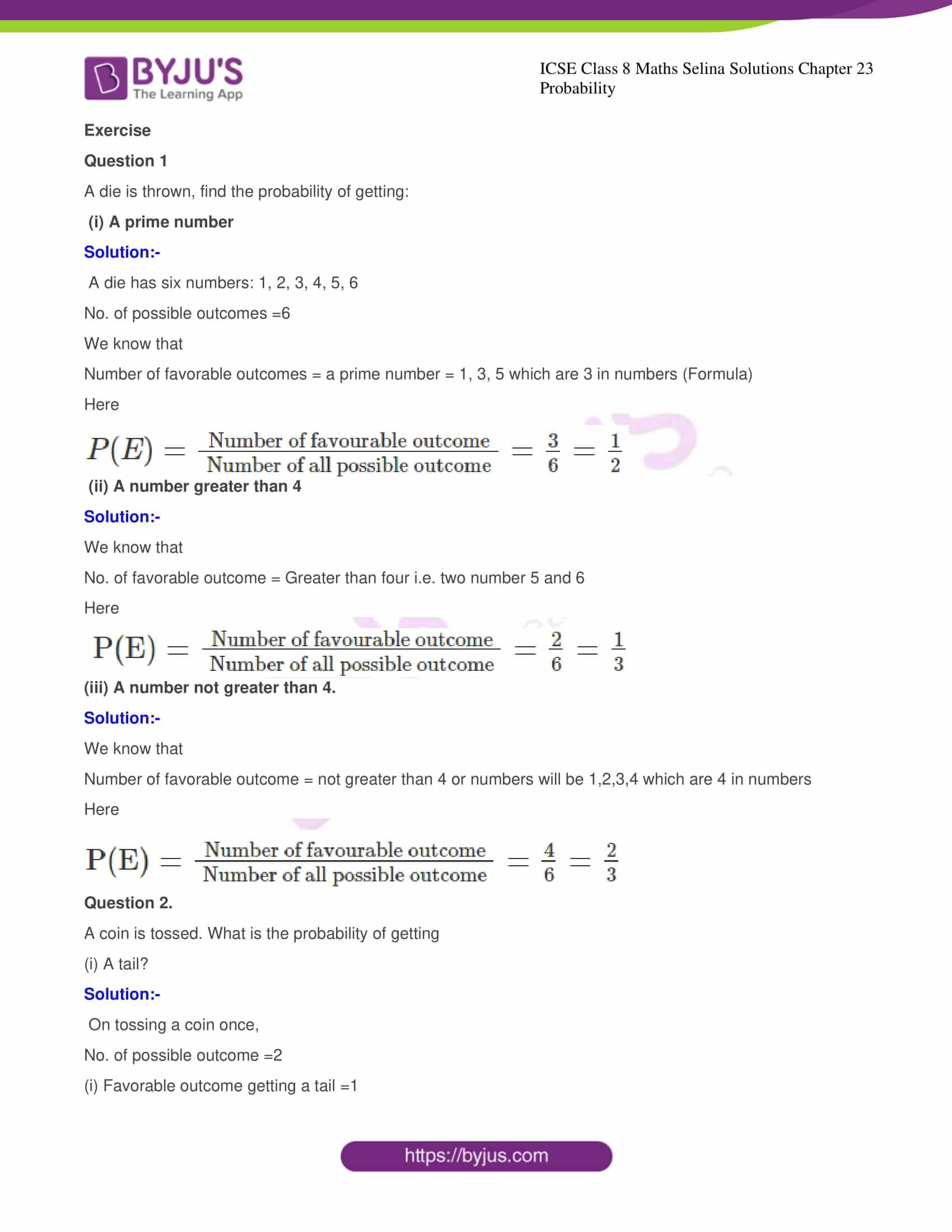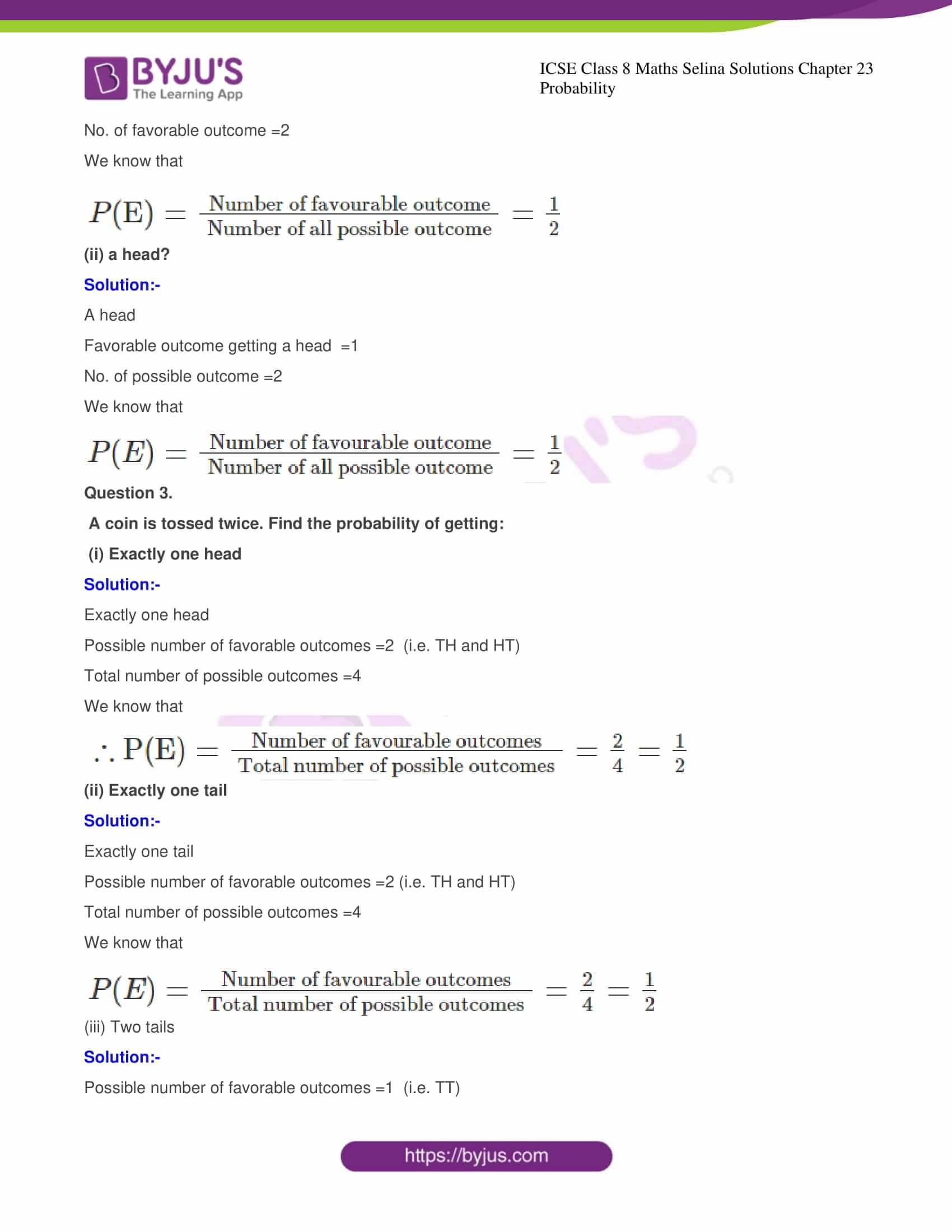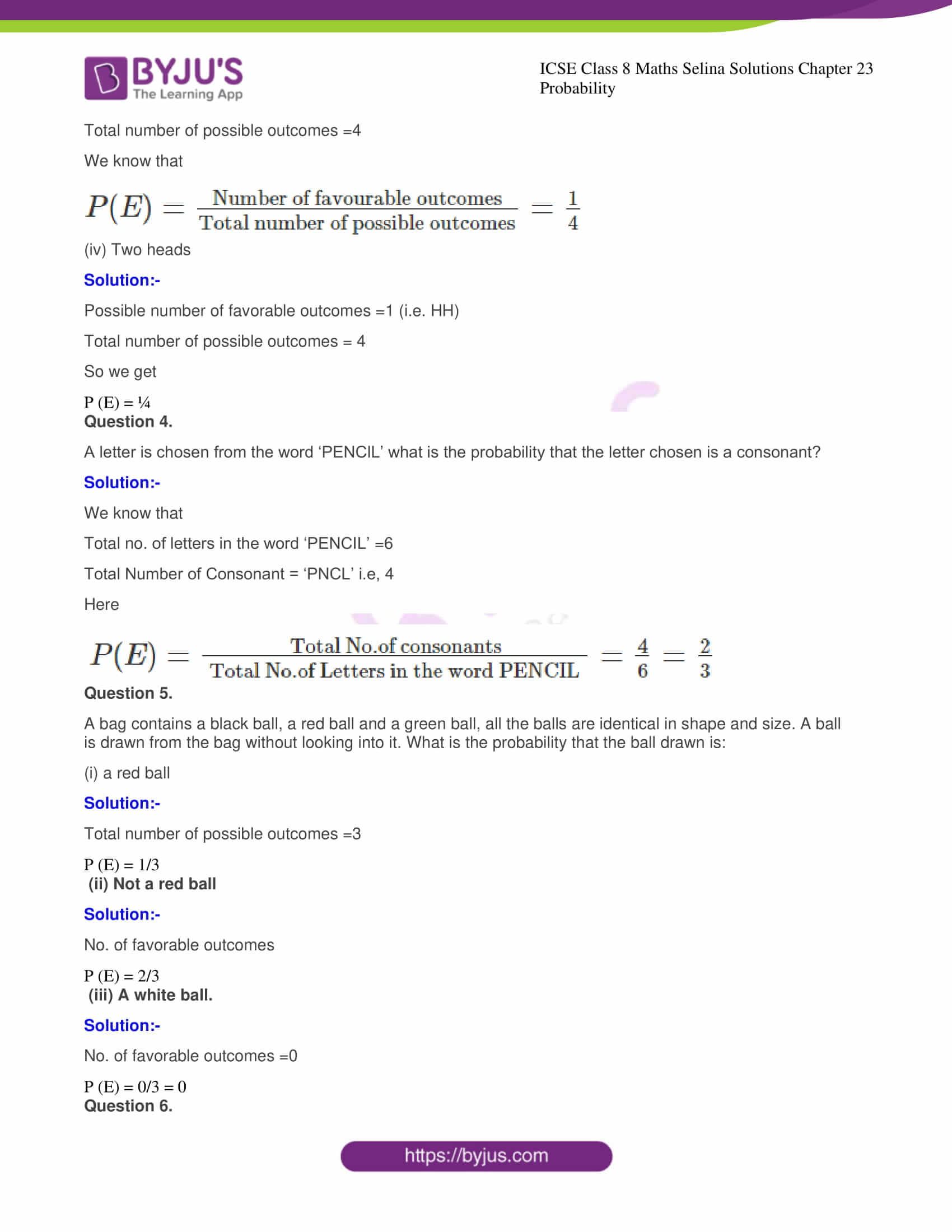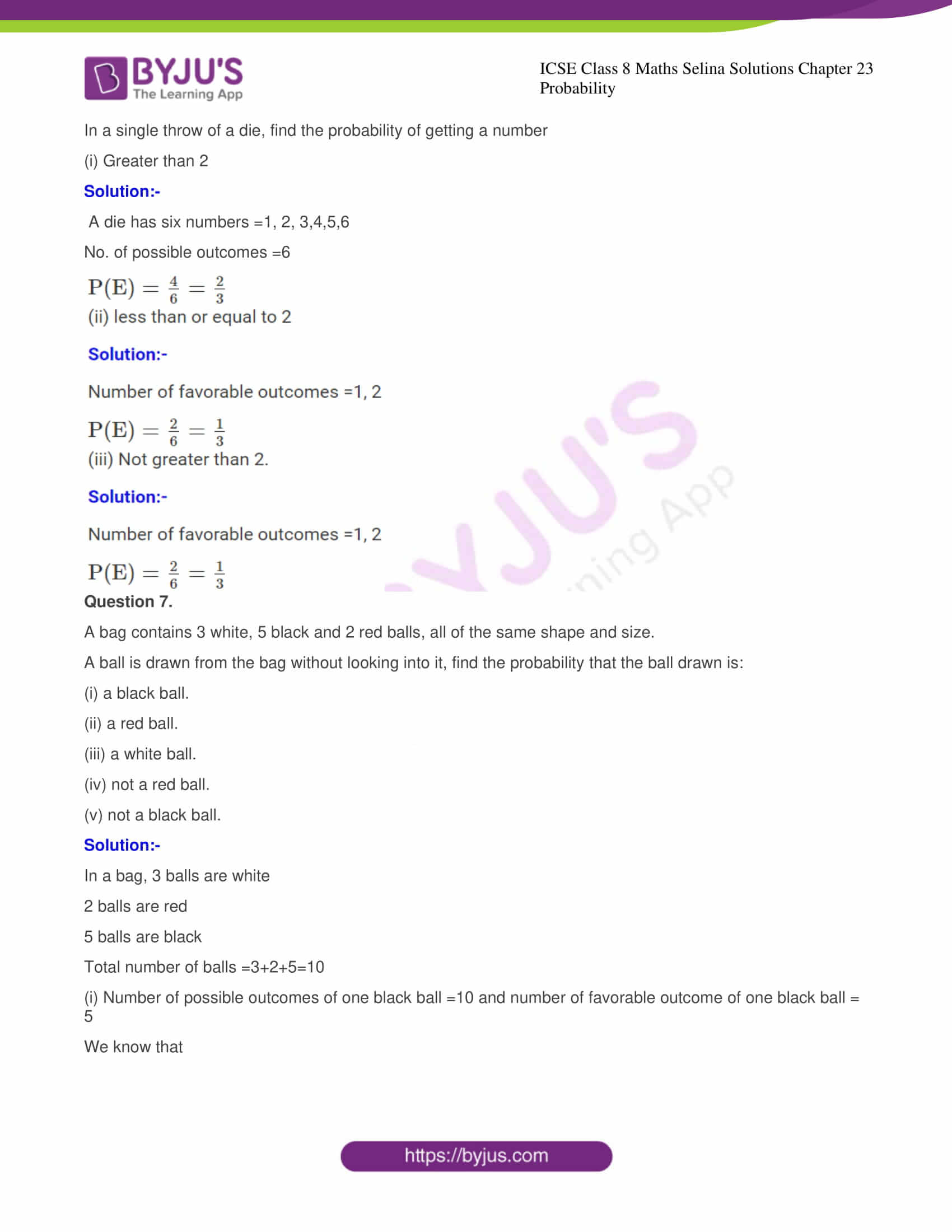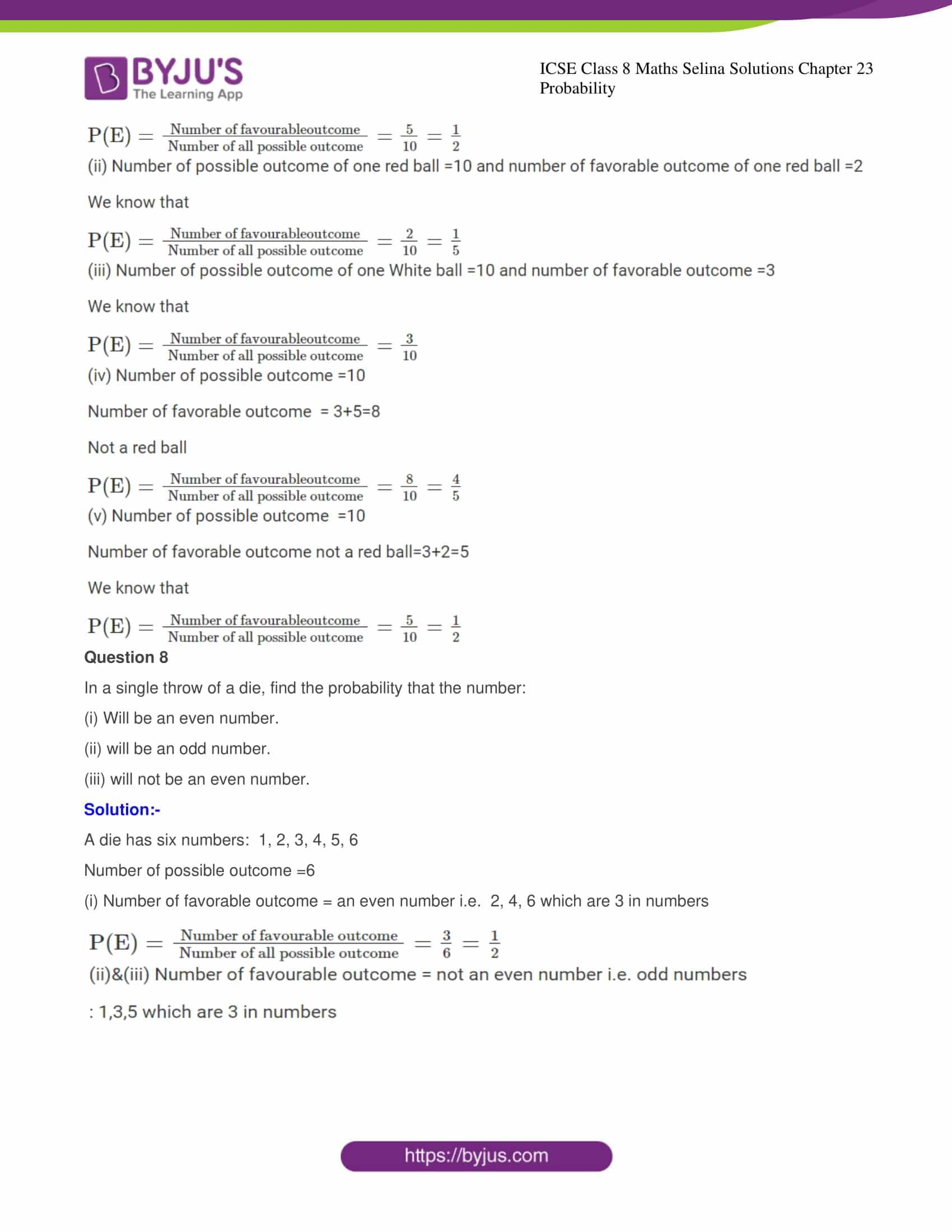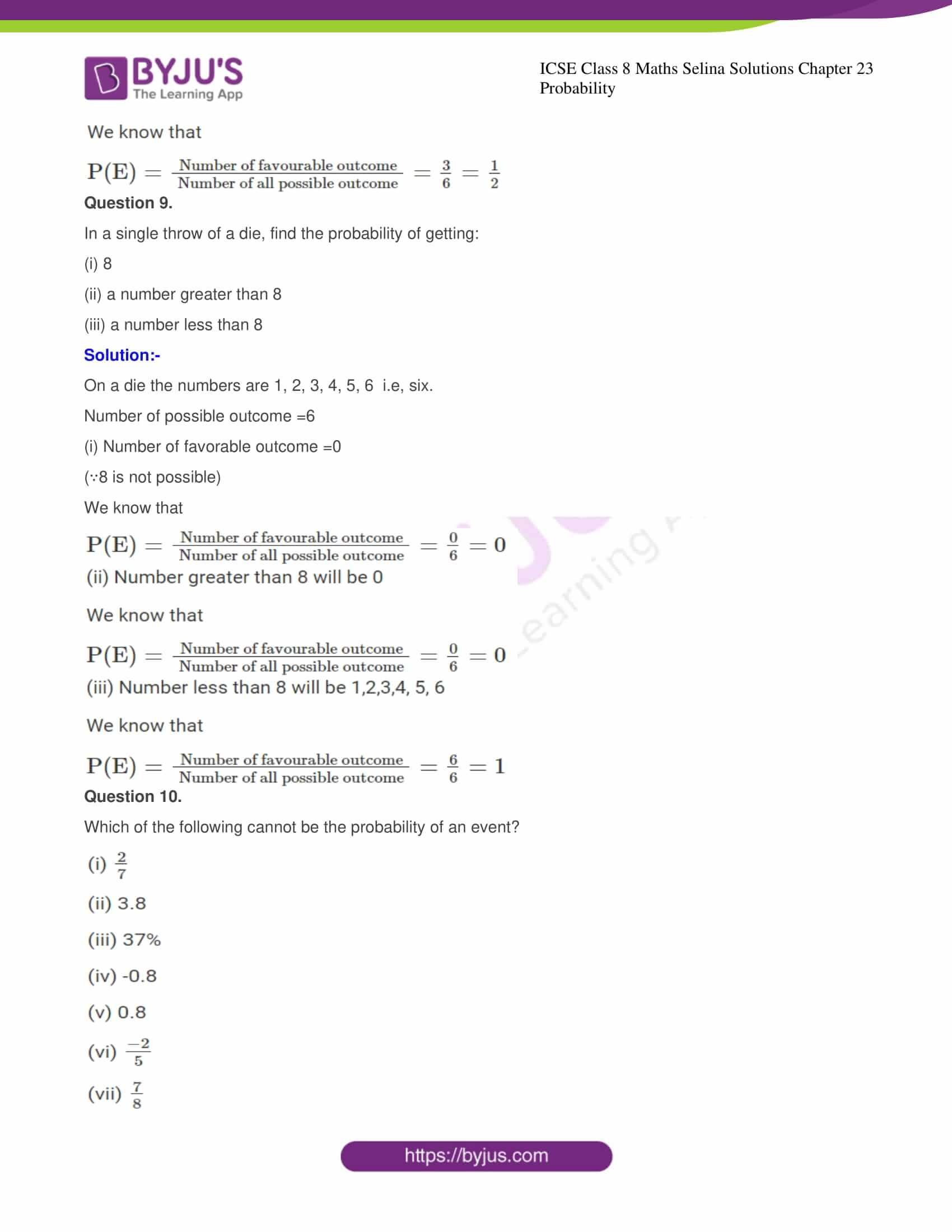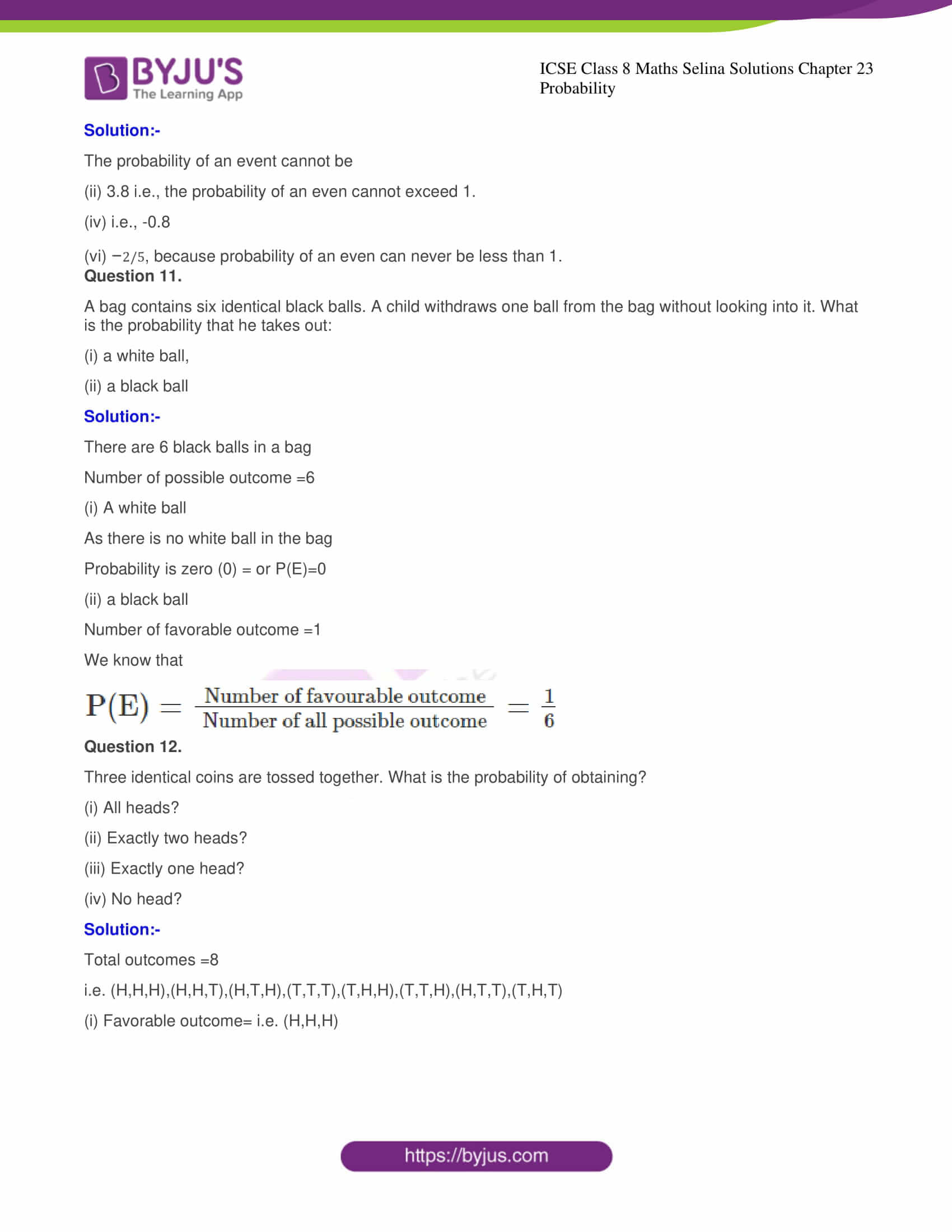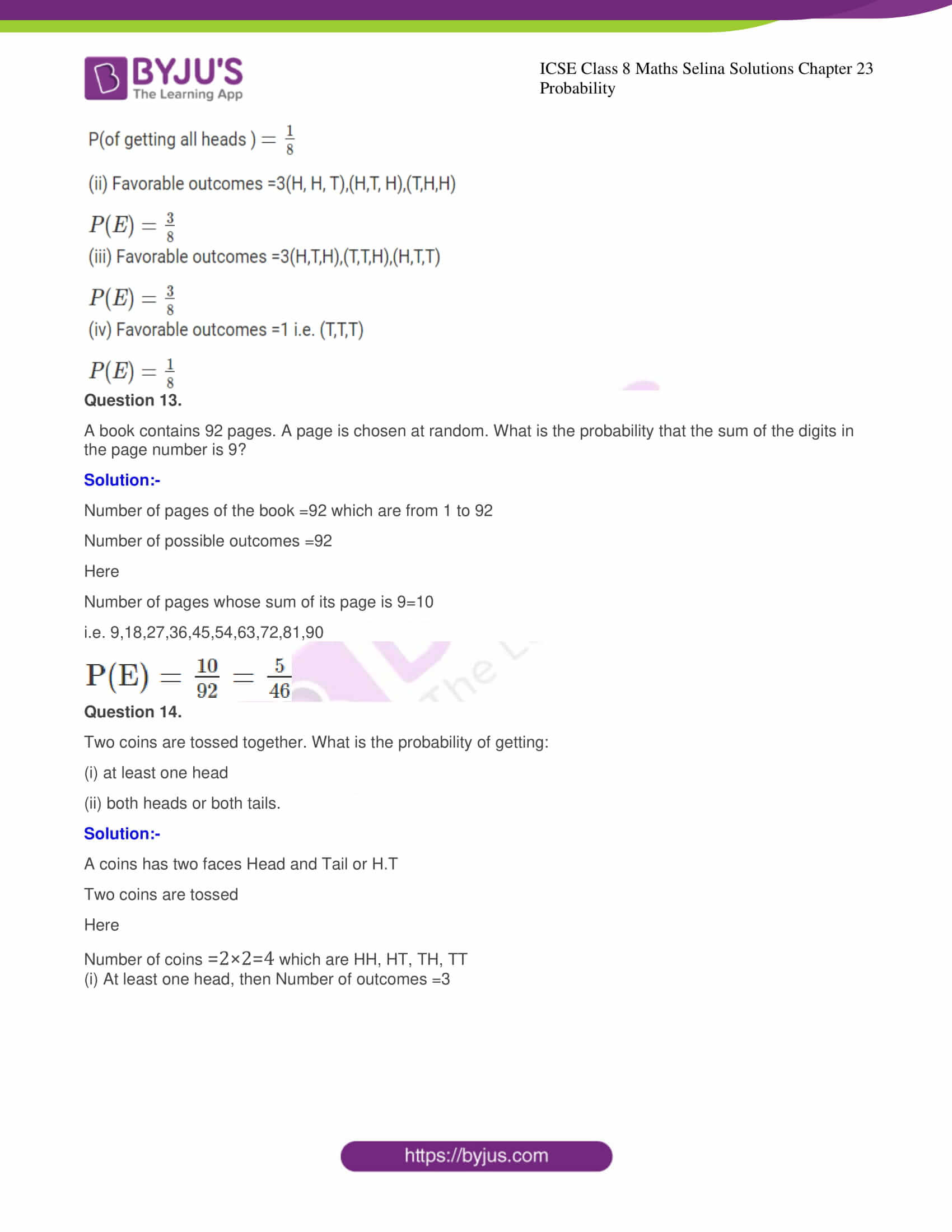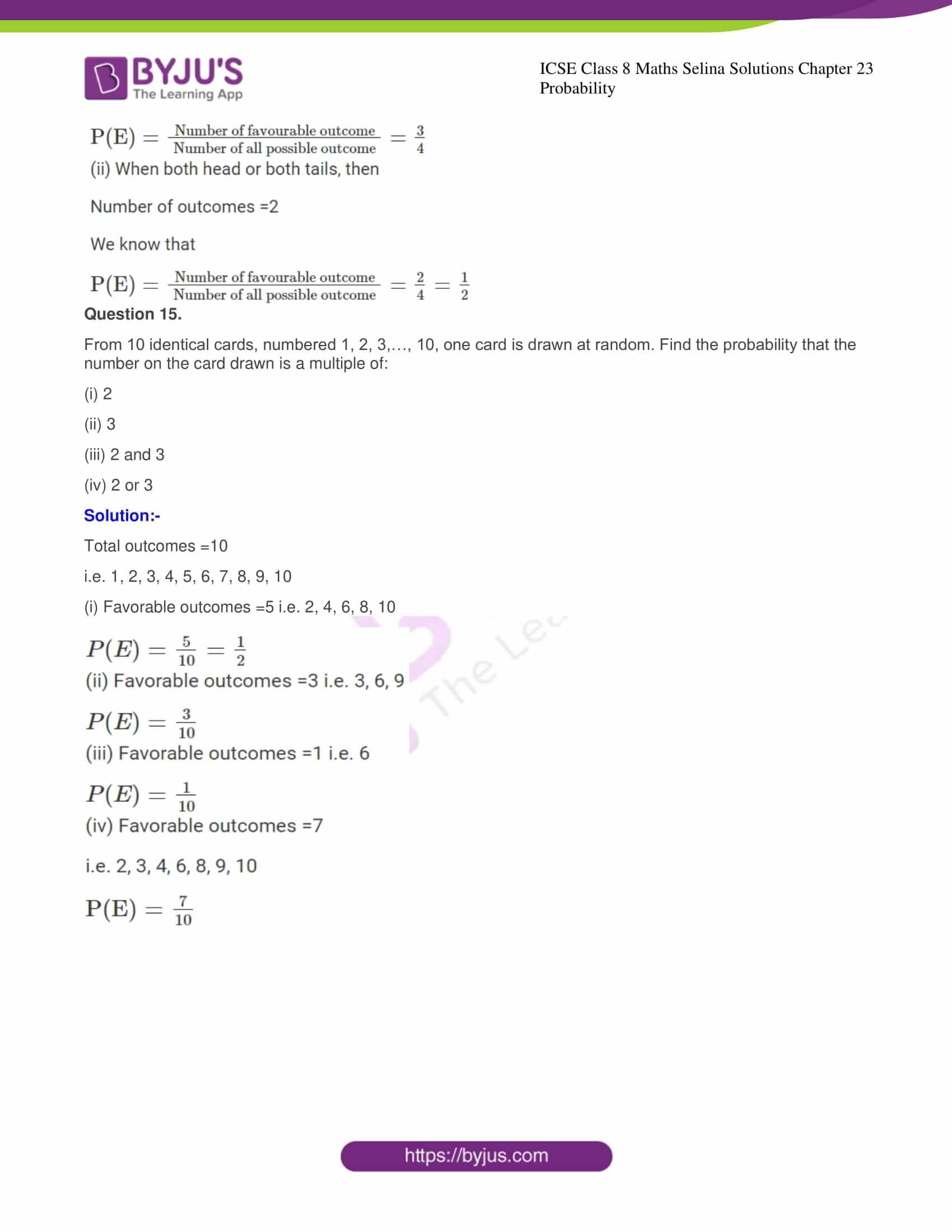## Access Selina Solutions Concise Maths Class 8 Chapter 23: Probability

Exercise

Question 1

A die is thrown, find the probability of getting:

(i) A prime number

Solution:-

A die has six numbers: 1, 2, 3, 4, 5, 6

No. of possible outcomes =6

We know that

Number of favorable outcomes = a prime number = 1, 3, 5 which are 3 in numbers (Formula)

Here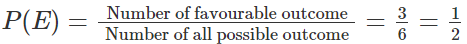(ii) A number greater than 4

Solution:-

We know that

No. of favorable outcome = Greater than four i.e. two number 5 and 6

Here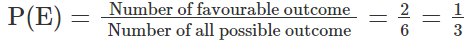(iii) A number not greater than 4.

Solution:-

We know that

Number of favorable outcome = not greater than 4 or numbers will be 1,2,3,4 which are 4 in numbers

Here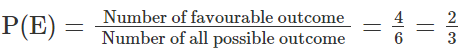Question 2.

A coin is tossed. What is the probability of getting

(i) A tail?

Solution:-

On tossing a coin once,

No. of possible outcome =2

(i) Favorable outcome getting a tail =1

No. of favorable outcome =2

We know that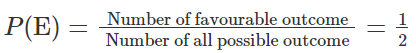Solution:-

Favorable outcome getting a head  =1

No. of possible outcome =2

We know that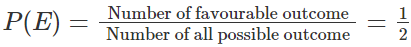Question 3.

A coin is tossed twice. Find the probability of getting:

Solution:-

Possible number of favorable outcomes =2  (i.e. TH and HT)

Total number of possible outcomes =4

We know that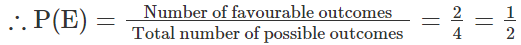(ii) Exactly one tail

Solution:-

Exactly one tail

Possible number of favorable outcomes =2 (i.e. TH and HT)

Total number of possible outcomes =4

We know that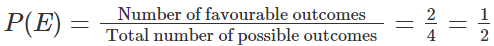(iii) Two tails

Solution:-

Possible number of favorable outcomes =1  (i.e. TT)

Total number of possible outcomes =4

We know that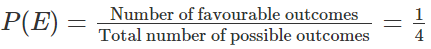Solution:-

Possible number of favorable outcomes =1 (i.e. HH)

Total number of possible outcomes = 4

So we get

P (E) = ¼

Question 4.

A letter is chosen from the word ‘PENClL’ what is the probability that the letter chosen is a consonant?

Solution:-

We know that

Total no. of letters in the word ‘PENCIL’ =6

Total Number of Consonant = ‘PNCL’ i.e, 4

Here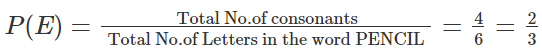Question 5.

A bag contains a black ball, a red ball and a green ball, all the balls are identical in shape and size. A ball is drawn from the bag without looking into it. What is the probability that the ball drawn is:

(i) a red ball

Solution:-

Total number of possible outcomes =3

P (E) = 1/3

(ii) Not a red ball

Solution:-

No. of favorable outcomes

P (E) = 2/3

(iii) A white ball.

Solution:-

No. of favorable outcomes =0

P (E) = 0/3 = 0

Question 6.

In a single throw of a die, find the probability of getting a number

(i) Greater than 2

Solution:-

A die has six numbers =1, 2, 3,4,5,6

No. of possible outcomes =6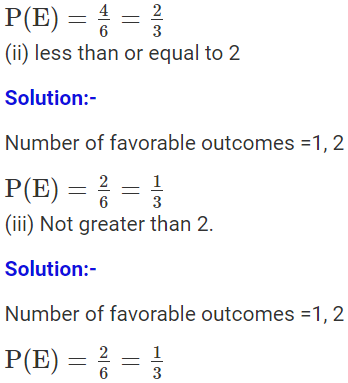Question 7.

A bag contains 3 white, 5 black and 2 red balls, all of the same shape and size.

A ball is drawn from the bag without looking into it, find the probability that the ball drawn is:

(i) a black ball.

(ii) a red ball.

(iii) a white ball.

(iv) not a red ball.

(v) not a black ball.

Solution:-

In a bag, 3 balls are white

2 balls are red

5 balls are black

Total number of balls =3+2+5=10

(i) Number of possible outcomes of one black ball =10 and number of favorable outcome of one black ball =5

We know that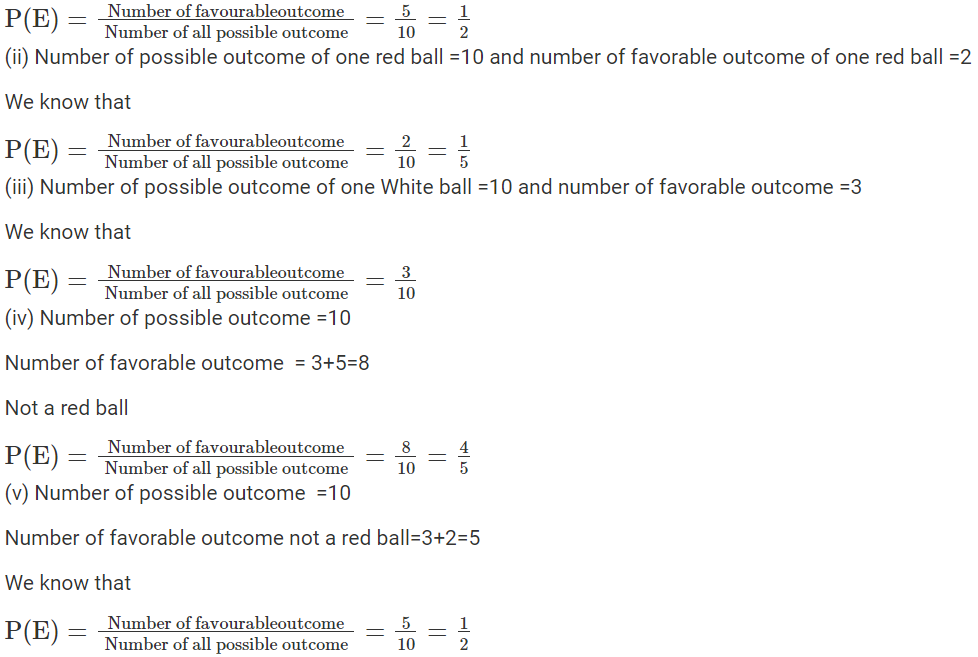Question 8

In a single throw of a die, find the probability that the number:

(i) Will be an even number.

(ii) will be an odd number.

(iii) will not be an even number.

Solution:-

A die has six numbers:  1, 2, 3, 4, 5, 6

Number of possible outcome =6

(i) Number of favorable outcome = an even number i.e.  2, 4, 6 which are 3 in numbers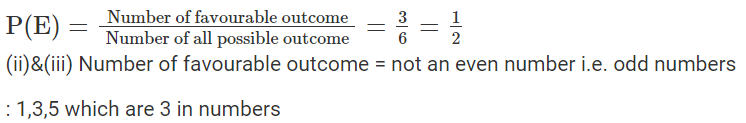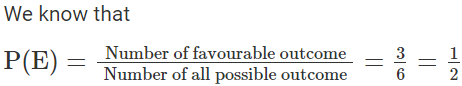Question 9.

In a single throw of a die, find the probability of getting:

(i) 8

(ii) a number greater than 8

(iii) a number less than 8

Solution:-

On a die the numbers are 1, 2, 3, 4, 5, 6  i.e, six.

Number of possible outcome =6

(i) Number of favorable outcome =0

(∵8 is not possible)

We know that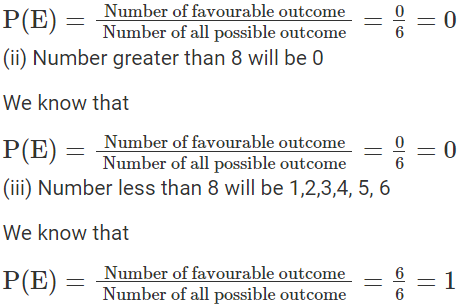Question 10.

Which of the following cannot be the probability of an event?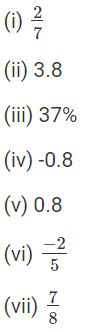Solution:-

The probability of an event cannot be

(ii) 3.8 i.e., the probability of an even cannot exceed 1.

(iv) i.e., -0.8

(vi) −2/5, because probability of an even can never be less than 1.

Question 11.

A bag contains six identical black balls. A child withdraws one ball from the bag without looking into it. What is the probability that he takes out:

(i) a white ball,

(ii) a black ball

Solution:-

There are 6 black balls in a bag

Number of possible outcome =6

(i) A white ball

As there is no white ball in the bag

Probability is zero (0) = or P(E)=0

(ii) a black ball

Number of favorable outcome =1

We know that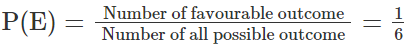Question 12.

Three identical coins are tossed together. What is the probability of obtaining?

Solution:-

Total outcomes =8

i.e. (H,H,H),(H,H,T),(H,T,H),(T,T,T),(T,H,H),(T,T,H),(H,T,T),(T,H,T)

(i) Favorable outcome= i.e. (H,H,H)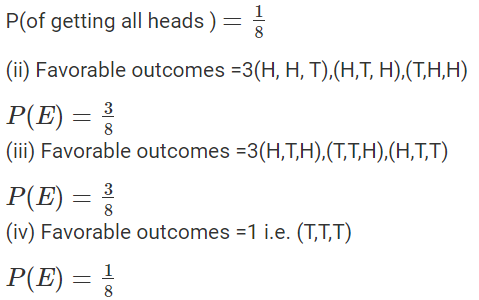Question 13.

A book contains 92 pages. A page is chosen at random. What is the probability that the sum of the digits in the page number is 9?

Solution:-

Number of pages of the book =92 which are from 1 to 92

Number of possible outcomes =92

Here

Number of pages whose sum of its page is 9=10

i.e. 9,18,27,36,45,54,63,72,81,90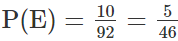Question 14.

Two coins are tossed together. What is the probability of getting:

(ii) both heads or both tails.

Solution:-

A coins has two faces Head and Tail or H.T

Two coins are tossed

Here

Number of coins =2×2=4 which are HH, HT, TH, TT

(i) At least one head, then Number of outcomes =3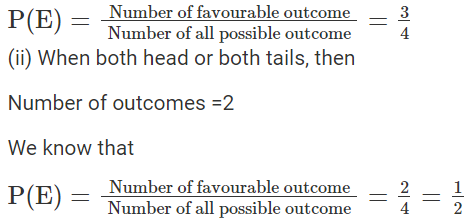Question 15.

From 10 identical cards, numbered 1, 2, 3,…, 10, one card is drawn at random. Find the probability that the number on the card drawn is a multiple of:

(i) 2

(ii) 3

(iii) 2 and 3

(iv) 2 or 3

Solution:-

Total outcomes =10

i.e. 1, 2, 3, 4, 5, 6, 7, 8, 9, 10

(i) Favorable outcomes =5 i.e. 2, 4, 6, 8, 10